# 1427 Mysterious Cat

This mysterious Halloween cat shares twelve clues that can help you solve its puzzle. Each clue is the products of two factors from 1 to 12 multiplied together. Will you be able to solve its mystery?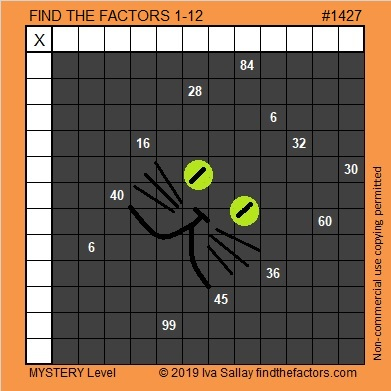Print the puzzles or type the solution in this excel file: 12 Factors 1419-1429

Now I’ll tell you a little bit about the puzzle number, 1427:

• 1427 is a prime number.
• Prime factorization: 1427 is prime.
• 1427 has no exponents greater than 1 in its prime factorization, so √1427 cannot be simplified.
• The exponent in the prime factorization is 1. Adding one to that exponent we get (1 + 1) = 2. Therefore 1427 has exactly 2 factors.
• The factors of 1427 are outlined with their factor pair partners in the graphic below.

How do we know that 1427 is a prime number? If 1427 were not a prime number, then it would be divisible by at least one prime number less than or equal to √1427. Since 1427 cannot be divided evenly by 2, 3, 5, 7, 11, 13, 17, 19, 23, 29 31, or 37, we know that 1427 is a prime number.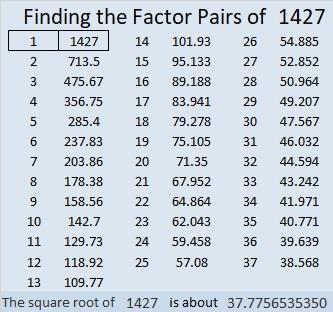1427 is 272 in BASE 25 because 2(25²) + 7(25) + 2(1) = 1427

# 1425 Jack O’Lantern

Most of my puzzles don’t have as many clues as this Jack O’lantern puzzle has. Those extra clues could make it easier to solve. On the other hand, some of the clues might still be tricky.Print the puzzles or type the solution in this excel file: 12 Factors 1419-1429

Here are some facts about the puzzle number, 1425:

• 1425 is a composite number.
• Prime factorization: 1425 = 3 × 5 × 5 × 19, which can be written 1425 = 3 × 5² × 19
• 1425 has at least one exponent greater than 1 in its prime factorization so √1425 can be simplified. Taking the factor pair from the factor pair table below with the largest square number factor, we get √1425 = (√25)(√57) = 5√57
• The exponents in the prime factorization are 1, 2, and 1. Adding one to each exponent and multiplying we get (1 + 1)(2 + 1)(1 + 1) = 2 × 3 × 2 = 12. Therefore 1425 has exactly 12 factors.
• The factors of 1425 are outlined with their factor pair partners in the graphic below.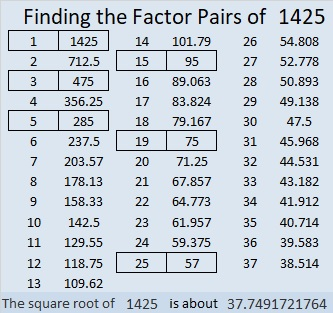1425 is the hypotenuse of TWO Pythagorean triples:
399-1368-1425 which is (7-24-25) times 57
855-1140-1425 which is (3-4-5) times 285

# 1424 Logic and Fear

Should this puzzle excite fear in you or anyone else? The logical answer is no, of course not. Logic can also help you to find the solution, so don’t be afraid, give it a try!Print the puzzles or type the solution in this excel file: 12 Factors 1419-1429

Finding the factors of 1424 shouldn’t bring fear to you either:

• 1424 is a composite number.
• Prime factorization: 1424 = 2 × 2 × 2 × 2 × 89, which can be written 1424 = 2⁴ × 89
• 1424 has at least one exponent greater than 1 in its prime factorization so √1424 can be simplified. Taking the factor pair from the factor pair table below with the largest square number factor, we get √1424 = (√16)(√89) = 4√89
• The exponents in the prime factorization are 4 and 1. Adding one to each exponent and multiplying we get (4 + 1)(1 + 1) = 5 × 2 = 10. Therefore 1424 has exactly 10 factors.
• The factors of 1424 are outlined with their factor pair partners in the graphic below.1424 is the sum of two squares:
32² + 20² = 1424

1424 is the hypotenuse of a Pythagorean triple:
624-1280-1424 calculated from 32² – 20², 2(32)(20), 32² + 20²

# 1423 Lollipop

Lollipops are candies that easily delight children. Will today’s lollipop-wannabe puzzle be a delight?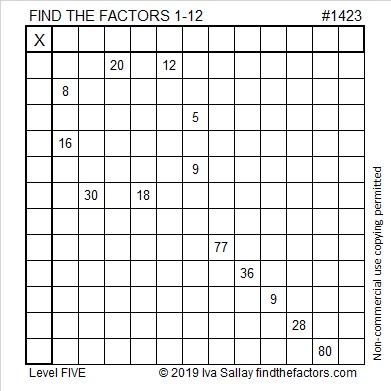Print the puzzles or type the solution in this excel file: 12 Factors 1419-1429

The puzzle number is 1423. Here are a few facts about that number:

• 1423 is a prime number.
• Prime factorization: 1423 is prime.
• 1423 has no exponents greater than 1 in its prime factorization, so √1423 cannot be simplified.
• The exponent in the prime factorization is 1. Adding one to that exponent we get (1 + 1) = 2. Therefore 1423 has exactly 2 factors.
• The factors of 1423 are outlined with their factor pair partners in the graphic below.How do we know that 1423 is a prime number? If 1423 were not a prime number, then it would be divisible by at least one prime number less than or equal to √1423. Since 1423 cannot be divided evenly by 2, 3, 5, 7, 11, 13, 17, 19, 23, 29 31, or 37, we know that 1423 is a prime number.

# 1422 Candy Corn Mystery

You can begin this candy corn puzzle easily enough, but the logic needed to solve it is a bit complicated. Good luck with this one!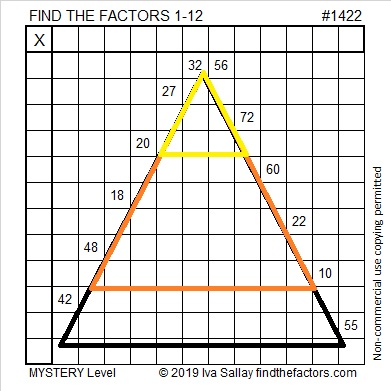Print the puzzles or type the solution in this excel file: 12 Factors 1419-1429

Now I’ll tell you some facts about the puzzle number, 1422:

• 1422 is a composite number.
• Prime factorization: 1422 = 2 × 3 × 3 × 79, which can be written 1422 = 2 × 3² × 79
• 1422 has at least one exponent greater than 1 in its prime factorization so √1422 can be simplified. Taking the factor pair from the factor pair table below with the largest square number factor, we get √1422 = (√9)(√158) = 3√158
• The exponents in the prime factorization are 1, 2, and 1. Adding one to each exponent and multiplying we get (1 + 1)(2 + 1)(1 + 1) = 2 × 3 × 2 = 12. Therefore 1422 has exactly 12 factors.
• The factors of 1422 are outlined with their factor pair partners in the graphic below.1422 is palindrome 1K1 in BASE 29 (K is 20 base 10)
because 1(29²) + 20(29¹) + 1(29º) = 1422.

# 1421 Square-shaped Sweet

This square-shaped puzzle is a Level 3 because you can start with clue 44 and work down the puzzle cell by cell to find the solution. You won’t get into a rhythm with this one, but logic will still help you to find the factors of the clues in order from top to bottom. That’s pretty sweet!Print the puzzles or type the solution in this excel file: 12 Factors 1419-1429

Now here are some sweet facts about the puzzle number 1421:

• 1421 is a composite number.
• Prime factorization: 1421 = 7 × 7 × 29, which can be written 1421 = 7² × 29
• 1421 has at least one exponent greater than 1 in its prime factorization so √1421 can be simplified. Taking the factor pair from the factor pair table below with the largest square number factor, we get √1421 = (√49)(√29) = 7√29
• The exponents in the prime factorization are 2 and 1. Adding one to each exponent and multiplying we get (2 + 1)(1 + 1) = 3 × 2 = 6. Therefore 1421 has exactly 6 factors.
• The factors of 1421 are outlined with their factor pair partners in the graphic below.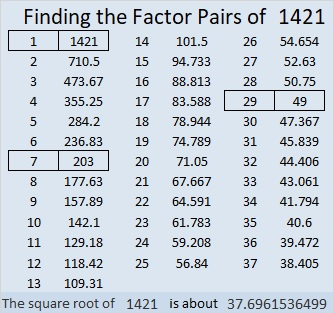1421 is also the hypotenuse of a Pythagorean triple:
980-1029-1421 which is (20-21-29) times 49.

# 1420 Eight-legged Creature

When you look at today’s puzzle, you might see a snowflake or perhaps an eight-legged creature. I hope you will also see lots of common factors that will help you solve this quick puzzle.Print the puzzles or type the solution in this excel file: 12 Factors 1419-1429

Here are some facts about the puzzle number, 1420:

• 1420 is a composite number.
• Prime factorization: 1420 = 2 × 2 × 5 × 71, which can be written 1420 = 2² × 5 × 71
• 1420 has at least one exponent greater than 1 in its prime factorization so √1420 can be simplified. Taking the factor pair from the factor pair table below with the largest square number factor, we get √1420 = (√4)(√355) = 2√355
• The exponents in the prime factorization are 2, 1, and 1. Adding one to each exponent and multiplying we get (2 + 1)(1 + 1)(1 + 1) = 3 × 2 × 2 = 12. Therefore 1420 has exactly 12 factors.
• The factors of 1420 are outlined with their factor pair partners in the graphic below.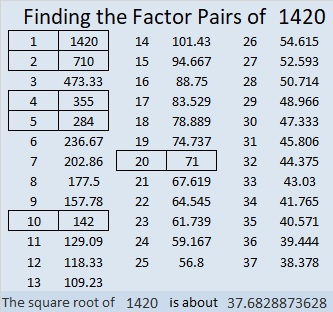1420 is the hypotenuse of a Pythagorean triple:
852-1136-1420 which is (3-4-5) times 284.

# 1419 and Level 1

This Level 1 Find the Factors 1-12 puzzle is as simple as clockwork. Can you find all the factors?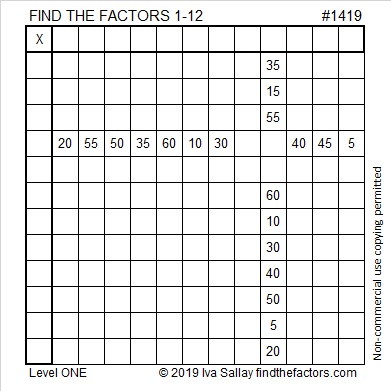Print the puzzles or type the solution in this excel file: 12 Factors 1419-1429

Now I’ll tell you a few things about the puzzle number, 1419:

• 1419 is a composite number.
• Prime factorization: 1419 = 3 × 11 × 43
• 1419 has no exponents greater than 1 in its prime factorization, so √1419 cannot be simplified.
• The exponents in the prime factorization are 1, 1, and 1. Adding one to each exponent and multiplying we get (1 + 1)(1 + 1)(1 + 1) = 2 × 2 × 2 = 8. Therefore 1419 has exactly 8 factors.
• The factors of 1419 are outlined with their factor pair partners in the graphic below.1419 is the difference of two squares in four different ways:
710² – 709² = 1419
238² – 235² = 1419
70² – 59² = 1419
38² – 5² = 1419

That last one means we are only 25 numbers away from the next perfect square, and that seems like clockwork, too.

1419 is only the second Zeisel number: (105 was the first.)
(4×1-1)(4×3-1)(4×11-1) = 3×11×43 =1419

# 1418 Challenge Puzzle

The 19 clues in this Find the Factors Challenge Puzzle are enough to find its unique solution. Can you find it?Print the puzzles or type the solution in this excel file: 10 Factors 1410-1418

Now I’ll write a few facts about the puzzle number, 1418:

• 1418 is a composite number.
• Prime factorization: 1418 = 2 × 709
• 1418 has no exponents greater than 1 in its prime factorization, so √1418 cannot be simplified.
• The exponents in the prime factorization are 1, and 1. Adding one to each exponent and multiplying we get (1 + 1)(1 + 1) = 2 × 2 = 4. Therefore 1418 has exactly 4 factors.
• The factors of 1418 are outlined with their factor pair partners in the graphic below.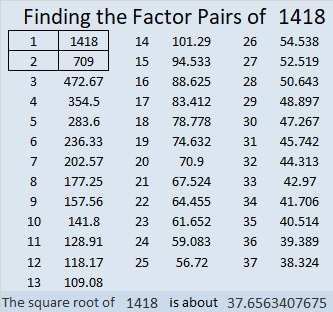1418 is the sum of two squares:
37² + 7² = 1418

518-1320-1418 calculated from 2(37)(7), 37² – 7², 37² + 7².
It is also 2 times (259-660-709)

# 1417 Mystery Puzzle

How hard is today’s puzzle? It’s a little harder just because I’m not telling what the level number is. Are you going to let that stop you from finding the unique solution? I hope not!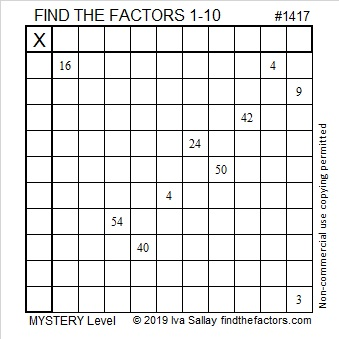Print the puzzles or type the solution in this excel file: 10 Factors 1410-1418

1417 is just the puzzle number, but in case you want to know something about it, here are some facts:

• 1415 is a composite number.
• Prime factorization: 1415 = 13 × 109
• 1415 has no exponents greater than 1 in its prime factorization, so √1415 cannot be simplified.
• The exponents in the prime factorization are 1, and 1. Adding one to each exponent and multiplying we get (1 + 1)(1 + 1) = 2 × 2 = 4. Therefore 1415 has exactly 4 factors.
• The factors of 1415 are outlined with their factor pair partners in the graphic below.1417 is the sum of two squares in two different ways:
29² + 24² = 1417
36² + 11² = 1417

1417 is the hypotenuse of FOUR Pythagorean triples:
265-1392-1417 calculated from 29² – 24², 2(29)(24), 29² + 24²
545-1308-1417 which is (5-12-13) times 109
780-1183-1417 which is 13 times (60-91-109)
792-1175-1417 calculated from 2(36)(11) , 36² – 11² , 36² + 11²